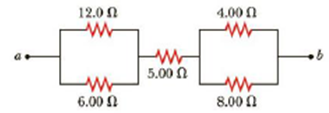Chapter 18, Problem 8P

Chapter
Section
Textbook Problem

Consider the combination of resistors shown in Figure P18.8. (a) Find the equivalent resistance between point a and b. (b) If a voltage of 35.0 V is applied between points a and b, find the current in each resistor.Figure P18.8

(a)

To determine
The equivalent resistance between the points a andb.

Explanation

Given Info:

The two parallel resistors is R1=12.0Ω and R2=6.00Ω .

Explanation:

Formula to calculate the equivalent resistance of the two parallel resistors is,

1RP1=1R1+1R2

• RP1 is the equivalent resistance for the parallel combination ,
• R1 and R2 is the two parallel resistors,

Rewrite the above relation as RP1 .

RP1=R1R2R1+R2

Substitute 12.0Ω for R1 and 6.00Ω for R2 .

RP1=(12.0Ω)(6.00Ω)12.0Ω+6.00Ω=4.00Ω

Thus, the equivalent resistance of the two parallel resistors is RP1=4.00Ω .

Formula to calculate the equivalent resistance of the two parallel resistors is,

1RP2=1R4+1R5

• RP2 is the equivalent resistance for the parallel combination ,
• R4 and R5 is the two parallel resistors,

Rewrite the above relation as RP2

(b)

To determine
The current through each resistor from the given figure P18.8.

Still sussing out bartleby?

Check out a sample textbook solution.

See a sample solution

The Solution to Your Study Problems

Bartleby provides explanations to thousands of textbook problems written by our experts, many with advanced degrees!

Get Started

The best and safest source of vitamin D for people in the United States is exposure to sunshine. T F

Nutrition: Concepts and Controversies - Standalone book (MindTap Course List)

What are the chemical differences between DNA and RNA?

Biology: The Dynamic Science (MindTap Course List)

Which of the following structures represent esters?

General, Organic, and Biological Chemistry